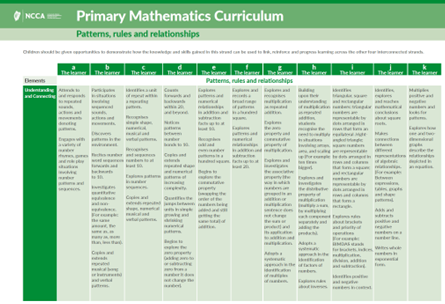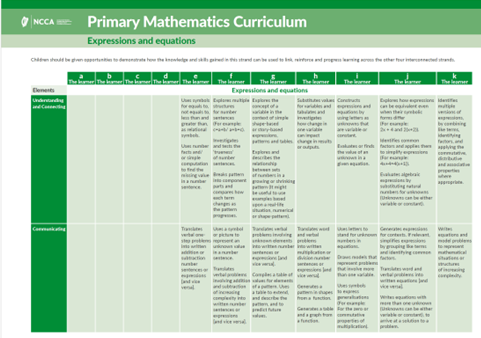NCCA uses cookies so that you have the best possible browsing experience on our website. If you agree that we can store and use cookies click 'Accept & Close'.Manage Cookies

# Algebra

Find below the Learning Outcomes, mathematical concepts and progression continua associated with the strand units of "Patterns, rules and relationships" and "Expressions and equations". This page will be updated with further support materials and video examples of children's learning in the coming weeks and months.

## Patterns, rules and relationships

### Stage 4

#### 5th & 6th Class Through appropriately playful and engaging learning experiences, children should be able to

explore, extend and create patterns and sequences.

identify and express relationships in patterns, including growing or shrinking shape patterns and number sequences.

identify rules that describe the structure of a pattern and use these rules to make predictions.

represent the relationships between quantities.

identify, explain and apply generalisations, including properties of operations, mathematical models and patterns.

represent mathematical structures in multiple ways, including verbal expressions, diagrams and symbolic representations.

## Mathematical Concepts

 A pattern is an arrangement of elements organised according to a defined structure or rule. A sequence is a list of objects or actions that follow an order. There are patterns and sequences to everyday routines and events, from which predictions can be made. Repeating patterns are made up of units of repeat and do not contain random elements. The zero property of addition and subtraction means that when you add zero to or subtract zero from a number it does not change the number. Quantitative change in growing and shrinking patterns is consistent and predictable. We can describe the structure of a growing or shrinking pattern, in word or number sentences, and use our description to predict future terms. When exploring the structure of growing or shrinking patterns, it is useful to determine what is changing and what is staying the same. The commutative property of addition means we can swap the order of the numbers being added and still get the same total. Properties of operations (e.g. zero, commutative) and patterns in numbers can be used to determine number facts we don’t know from number facts we do know. Examples include doubles & near-doubles, inverses, adding 10, odd and even numbers. Number patterns tell the story of relationships between quantities. The relationship between elements of a pattern can be expressed using word or number sentences. Representations can be used to show and explore the relationships between quantities.  Representing scenarios, including patterns, in words, number sentences, diagrams and graphs can be useful to solve problems or to determine values for unknown or future events. A geometric sequence of numbers is based upon multiplication or division. Each consecutive number is found by multiplying the preceding number by a common multiplier. The associative property states that when three or more numbers are added or multiplied, the sum or product remains the same regardless of how the numbers are grouped. The distributive property means that complex multiplication and division equations can be simplified by breaking one (in the case of the dividend) or both numbers down into smaller parts. The structure of a pattern, or the property of operations, can be described succinctly by a mathematical expression, for example, the commutative property: a+b=b+a. Describing a real-life situation using words or symbols can be useful to solve problems or to determine values for unknown or future events. An integer is a whole number that can be positive, negative, or zero. Whole numbers can be expressed in standard form, exponential form or be written as a product of factors. A square number is what we get after multiplying an integer by itself. The square root of a number identifies what must be squared to get the number.

## Progression Continuum

Click on the image to access the progression continuum for the strand unit of 'Patterns rules, and relationships'### Stage 4

#### 5th & 6th Class Through appropriately playful and engaging learning experiences, children should be able to

interpret the meaning of symbols or pictures in number sentences.

represent and express problems with known and unknown values in different ways to include the use of appropriate letter-symbols or words.

articulate, represent and solve mathematical situations through the use of expressions and equations that include letter-symbols.

## Mathematical Concepts

 Real-life situations can be expressed using manipulatives, diagrams, and word and number sentences. An equals sign (=) conveys equality, whereas ≠, < and > convey inequality. In number sentences (equations), symbols can stand for a request to do something (+, -, x, ÷), they can express a relationship (=, <, >, ≠), or they can be something that is unknown or varies. In a number sentence, number facts can be applied to help find an unknown value. When expressing real-life situations, symbols can be used to represent an unknown, a quantity that varies (variable), or every number (the general case). Real-life situations and functions can be represented in a variety of forms, including numbers, words, symbols and tables. A function is a special relationship where each input has exactly one output. There are always three main parts; the input, the functional relationship and the output. Representing the structure of a function using words, symbols, graphs, tables or diagrams is useful to identify outputs for given inputs and vice versa. A real-life situation can be represented by an expression or a series of expressions. An expression may contain more than one unknown or variable. Each unknown or variable must be represented by a dedicated symbol. When generating an expression to represent a real-life situation, it can be possible and useful to ‘simplify’ a long or complex expression. ‘Solving’ an equation consists of determining which value(s) for a given symbol make(s) the equation true.

## Progression Continuum

Click on the image to access the progression continuum for the strand unit of 'Expressions and equations'